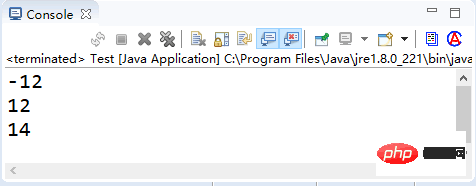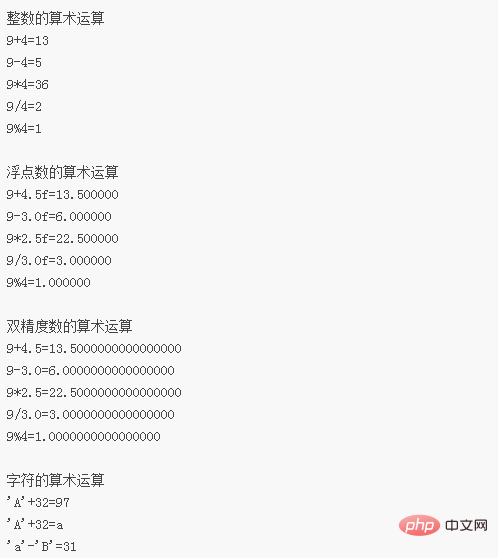# Java中的%是什么意思Java中的%是取余的意思，是一个算术运算符，可实现求余运算，进行除法运算并获取余数。

```a%b=a-(a/b)*b 5%3=5-(5/3)*3=2 5%-3=5-(5/-3)*-3=2 -5%3=-5-(-5/3)*3=-2 -5%-3=-5-(-5/-3)*-3=-2登录后复制```

`a%b=a-(b*q)`，这里`q=int(a/b)`

```5.2%3.1=5.2-1*3.1=2.1 5.2%-3.1=5.2-(-1)*(-3.1)=2.1 -5.2%3.1=-5.1-(-1)*3.1=-2.1 -5.2%-3.1=-5.1-(-1)*(-3.1)=-2.1登录后复制```

Java 中的算术运算符主要用来组织数值类型数据的算术运算，按照参加运算的操作数的不同可以分为一元运算符和二元运算符。

- 取反符号取反运算b=-a
++自加一先取值再加一，或先加一再取值a++ 或 ++a
--自减一先取值再减一，或先减一再取值a-- 或 --a

```int a = 12; System.out.println(-a); int b = a++; System.out.println(b); b = ++a; System.out.println(b);登录后复制```Java 语言中算术运算符的功能是进行算术运算，除了经常使用的加（+）、减（-）、乘（*）和除（\）外，还有取模运算（％）。加（+）、减（-）、乘（*）、除（\）和我们平常接触的数学运算具有相同的含义。具体说明参见表 2。

+求 a 加 b 的和，还可用于 String 类型，进行字符串连接操作a + b
-求 a 减 b 的差a - b
*求 a 乘以 b 的积a * b
/

%

```int a = 4, b = 2, c = 3; int d = a * (b + c) % c;登录后复制```

• `int x=2,y=1;`表达式 y/x 的结果是 0。

• `float x=2.0f; int y=1;` 表达式 y/x 的结果是 0.5。

【例1】编写一个程序，输出不同类型的两个数，执行相加、相减、相乘、相除和求余后输入结果。

```public static void main(String[] args) { float f1 = 9 % 4;// 保存取余后浮点类型的结果 double da = 9 + 4.5; // 双精度加法 double db = 9 - 3.0; // 双精度减法 double dc = 9 * 2.5; // 双精度乘法 double dd = 9 / 3.0; // 双精度除法 double de = 9 % 4; // 双精度取余 System.out.println("整数的算术运算"); // 整数的加、减、乘、除和取余 System.out.printf("9+4=%d \n", 9 + 4); System.out.printf("9-4=%d \n", 9 - 4); System.out.printf("9*4=%d \n", 9 * 4); System.out.printf("9/4=%d \n", 9 / 4); System.out.printf("9%%4=%d \n", 9 % 4); System.out.println("\n浮点数的算术运算"); // 浮点数的加、减、乘、除和取余 System.out.printf("9+4.5f=%f \n", 9 + 4.5f); System.out.printf("9-3.0f=%f \n", 9 - 3.0f); System.out.printf("9*2.5f=%f \n", 9 * 2.5f); System.out.printf("9/3.0f=%f \n", 9 / 3.0f); System.out.printf("9%%4=%f \n", f1); System.out.println("\n双精度数的算术运算"); // 双精度数的加、减、乘、除和取余 System.out.printf("9+4.5=%4.16f \n", da); System.out.printf("9-3.0=%4.16f \n", db); System.out.printf("9*2.5=%4.16f \n", dc); System.out.printf("9/3.0=%4.16f \n", dd); System.out.printf("9%%4=%4.16f \n", de); System.out.println("\n字符的算术运算"); // 对字符的加法和减法 System.out.printf("'A'+32=%d \n", 'A' + 32); System.out.printf("'A'+32=%c \n", 'A' + 32); System.out.printf("'a'-'B'=%d \n", 'a' - 'B'); }登录后复制```• 相关标签：取余 % 运算符 java
• 相关文章

相关视频